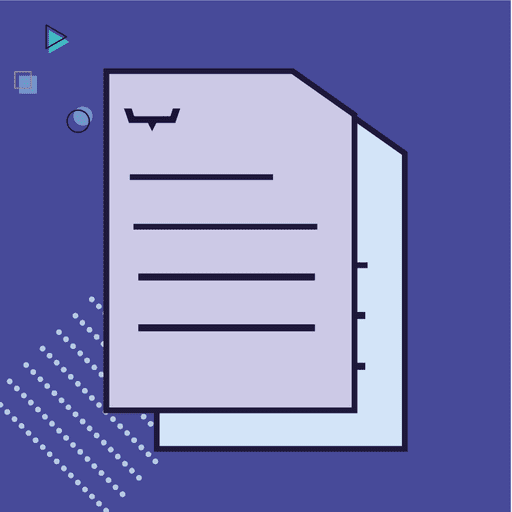#daysBetween / weeksBetween / monthBetween / yearsBetweenThis article explains how you can use time expressions to calculate the number of days, weeks, months or years between two instances. It also gives you example knowledge maps to explore time expressions inside the Rainbird Studio.The expressions discussed in this section are used by Rainbird to calculate the days/weeks/months or years between two dates instances that have either been created at runtime or that exist in the knowledge map.Click on an expression below for more information and for an example model that uses that expression:daysBetween: calculates the number of complete days between two datesweeksBetween: calculates the number of complete weeks (every 7 days) between two datesmonthsBetween: calculates the number of complete months between two datesyearsBetween: calculates the number of complete years between two datesdaysBetween / weeksBetween / monthBetween / yearsBetween - Downloadable Model0 followers0 questions1 post

You will need to register to be able to post or comment on a topic.

When viewing the knowledge map generated by the .rbird file below, please see the list below for which query you will need to run to see each date expression in action:

• daysBetween - 'has days remaining'
• weeksBetween -... (More)
Article
Library

This article will demonstrate how to build a map that uses the yearsBetween() expression to calculate how many years have passed since the beginning of the millennium. The map will count the number of years between the date the query... (More)

Article
Library

This article will demonstrate how to build a map that will use the monthsBetween() expression to count the number of months remaining until a pregnant person's due date.

Creating Concepts & Relationships

Create the following concepts:

1 String Concept... (More)

Article
Library

This article will demonstrate how to build a map that uses the weeksBetween() expression to calculate the remaining weeks until Christmas.

Creating Concepts & Relationships

Create the following concepts:

1 string concept – ‘Person’

1 number concept – ‘Christmas’... (More)